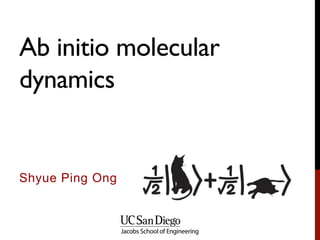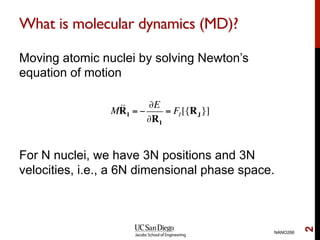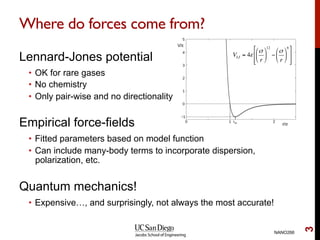Seu SlideShare está sendo baixado. ×

# NANO266 - Lecture 13 - Ab initio molecular dyanmics

Anúncio
Anúncio
Anúncio
Anúncio
Anúncio
Anúncio
Anúncio
Anúncio
Anúncio
Anúncio
AnúncioCarregando em…3
×

1 de 23 Anúncio

# NANO266 - Lecture 13 - Ab initio molecular dyanmics

UCSD NANO 266 Quantum Mechanical Modelling of Materials and Nanostructures is a graduate class that provides students with a highly practical introduction to the application of first principles quantum mechanical simulations to model, understand and predict the properties of materials and nano-structures. The syllabus includes: a brief introduction to quantum mechanics and the Hartree-Fock and density functional theory (DFT) formulations; practical simulation considerations such as convergence, selection of the appropriate functional and parameters; interpretation of the results from simulations, including the limits of accuracy of each method. Several lab sessions provide students with hands-on experience in the conduct of simulations. A key aspect of the course is in the use of programming to facilitate calculations and analysis.

UCSD NANO 266 Quantum Mechanical Modelling of Materials and Nanostructures is a graduate class that provides students with a highly practical introduction to the application of first principles quantum mechanical simulations to model, understand and predict the properties of materials and nano-structures. The syllabus includes: a brief introduction to quantum mechanics and the Hartree-Fock and density functional theory (DFT) formulations; practical simulation considerations such as convergence, selection of the appropriate functional and parameters; interpretation of the results from simulations, including the limits of accuracy of each method. Several lab sessions provide students with hands-on experience in the conduct of simulations. A key aspect of the course is in the use of programming to facilitate calculations and analysis.

Anúncio
Anúncio

### NANO266 - Lecture 13 - Ab initio molecular dyanmics

1. 1. Ab initio molecular dynamics Shyue Ping Ong
2. 2. What is molecular dynamics (MD)? Moving atomic nuclei by solving Newton’s equation of motion For N nuclei, we have 3N positions and 3N velocities, i.e., a 6N dimensional phase space. NANO266 2 M!!RI = − ∂E ∂RI = FI [{RJ}]
3. 3. Where do forces come from? Lennard-Jones potential •  OK for rare gases •  No chemistry •  Only pair-wise and no directionality Empirical force-fields •  Fitted parameters based on model function •  Can include many-body terms to incorporate dispersion, polarization, etc. Quantum mechanics! •  Expensive…, and surprisingly, not always the most accurate! NANO266 3 VLJ = 4ε σ r ! " # \$ % & 12 − σ r ! " # \$ % & 6( ) * * + , - -
4. 4. 2013 Nobel Prize in Chemistry NANO266 4
5. 5. Computational Experiment NANO266 5 Initialize positions and velocities Compute forces Determine new positions Calculate thermodynamic averages Repeat until time is reached
6. 6. Initialization 2nd order PDF -> Initial positions and velocities Positions •  Reasonable guess based on structure •  No overlap / short atomic distances Velocities •  Small initial velocity •  Steadily increase temperature (velocity scaling) NANO266 6 M!!RI = − ∂E ∂RI = FI [{RI}]
7. 7. Maxwell-Boltzmann distribution NANO266 7 f (v) = m 2πkT ! " # \$ % & 3 4πv2 e − mv2 2kT
8. 8. Ensembles Microcanonical – N, V, E Canonical – N, V, T •  Temperature control achieved via thermostats •  Examples: velocity rescaling, Andersen thermostat, Nose-Hoover thermostat, Langevin dynamics, etc. NANO266 8
9. 9. Integrating equations of motion –Verlet algorithm Key properties of Verlet algorithm •  Errors do not accumulate •  Energy is conserved •  Simulations are stable NANO266 9 M!!RI = FI [{RJ}] !!RI = 1 MI FI [{RJ}] [RI (t + Δt)− RI (t)]−[RI (t)− RI (t − Δt)] (Δt)2 = 1 MI FI [{RJ}] RI (t + Δt) = 2RI (t)+ RI (t − Δt)+ (Δt)2 MI FI [{RJ (t)}]
10. 10. Flavors of quantum MD Born-Oppenheimer •  Electrons stay in instantaneous ground state as nuclei move •  Perform electronic SCF at each time step •  Compute forces (Hellman-Feynman theorem) •  Move nuclei Car-Parrinello •  Treat ions and electrons as one unified system •  Accomplished with fictitious kinetic energy for electrons NANO266 10 Car, R.; Parrinello, M. Unified approach for molecular dynamics and density-functional theory, Phys. Rev. Lett., 1985, 55, 2471–2474, doi: 10.1103/PhysRevLett.55.2471.
11. 11. Coupled equations of motion The CP Lagrangian Equations of motion NANO266 11 L = 1 2 (2µ) i=1 N ∑ dr !ψi (r) 2 ∫ + MI I=1 NI ∑ !!RI − E[ψi,r]+ Λij drψi * (r)ψj (r)−δij∫% & ' ( ij ∑ Imposes orthonormality of electronic states µ !!ψi (r,t) = −Hψj (r)+ Λikψk (r,t) k ∑ MI !!RI = − ∂E ∂RI
12. 12. Comparison of BO vs CP MD NANO266 12 CP Avoids need for costly SCF iteration of electrons at each step Time step required is smaller BO Solves Schrodinger equation at each step Time step can be longer
13. 13. General procedure for MD simulation NANO266 13
14. 14. Applications of MD Thermodynamics from ensemble averages Real-time evolution •  Reactions •  Interaction of molecules on surfaces •  Trajectories of ions Ground-state structures •  Geometry optimization is in fact a form of MD. •  For complex structures (e.g., low symmetry systems, interfaces, liquids, amorphous solids, etc.), MD can be used to determine low- energy structures NANO266 14 Nattino, F.; Ueta, H.; Chadwick, H.; Reijzen, M. E. Van; Beck, R. D.; Jackson, B.; Hemert, M. C. Van; Kroes, G. Ab Initio Molecular Dynamics Calculations versus Quantum-State- Resolved Experiments on CHD 3 + Pt(111): New Insights into a Prototypical Gas − Surface Reaction, J. Phys. Chem. Lett., 2014, 5, 1294–1299, doi:10.1021/jz500233n.
15. 15. Thermodynamic averages Under ergodic hypothesis Examples of averages •  Energy (potential, kinetic, total) •  Temperature •  Pressure •  Mean square displacements (diffusion) •  Radial distribution function NANO266 15 A = Aie−βEi (r,p) i ∑ e−βEi (r,p) i ∑ = lim T→∞ 1 T A(t)dt 0 T ∫
16. 16. Correlation Functions from MD Pair distribution function Time correlation function NANO266 16 g(r) = V N2 δ(r − rij) j≠i ∑ i ∑ Einstein relation (valid at large t) D = 1 3 dt v(t)v(0) 0 ∞ ∫ Example: Diffusion coefficient D = 1 2dt (r(t)− r(0))22tγ = (A(t)− A(0))2 γ = dt !A(t) !A(0) 0 ∞ ∫
17. 17. Structure ofAmorphous InP NANO266 17 Lewis, L.; De Vita, A.; Car, R. Structure and electronic properties of amorphous indium phosphide from first principles, Phys. Rev. B, 1998, 57, 1594–1606, doi:10.1103/PhysRevB.57.1594.
18. 18. Lithium hydroxide phase transition under high